# Evaluation

## Comparison of CFD Calculations with Experiments

This section is organized as follows. First, results of some sensitivity studies are presented and briefly discussed. These include evaluation of such effects as span-size of the domain, compressibility, time sample used for computing the mean flow and turbulent statistics, and numerical dissipation of the method used. Then, a comparison with the experimental data is shown for the main body of simulations carried out within the ATAAC project with the use of the physical and computational problem setups outlined in the Test Case section.

### RESULTS OF SENSITIVITY STUDIES

#### Effect of span size of domain

As mentioned in the Test Case Experiments section above, the aspect ratio of the CT configuration Lz/ D in the BART facility is equal to 12.4. Strictly speaking this demands carrying out simulations exactly at this value of Lz/ D and imposing no-slip boundary conditions on the floor and ceiling of the test section (see Figure 2). However such simulations would be very expensive. Considering this and, also, recommendations of the BANC-I Workshop based on simulations at different Lz/ D with periodic boundary conditions in the spanwise directions, most of the simulations in the ATAAC project were performed at Lz/ D = 3 assuming spanwise periodicity. In order to get an idea on how strong the effect of such a simplification could be, NTS conducted a series of simulations at different Lz/ D. Some results of these simulations are presented below 

Figure 4 compares flow visualisations from the SA DDES carried out in the "mandatory" (Lz/ D = 3) and the widest of the considered domains (Lz/ D = 16) in the form of instantaneous isosurface of the magnitude of the second eigenvalue of the velocity gradient tensor or "swirl" quantity, λ2. The figure is reassuring in the sense that it visibly displays that the narrow-domain simulation resolves not only fine-grained turbulent eddies but also large, nearly coherent, structures and exhibits all the complex flow features observed in the visualization of the wide-domain simulation, except for the initial region of the free shear-layer separated from the upstream cylinder, where a noticeable difference between the two flow-visualizations is observed.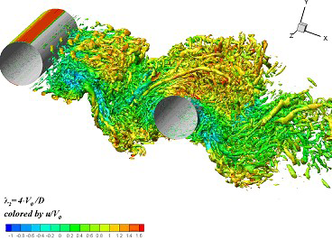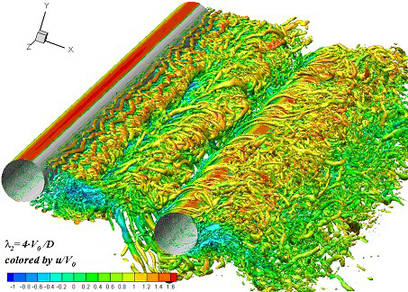Figure 4: Isosurface of λ2 = 4.0(U0 /D ) from incompressible SA DDES at Lz = 3D and 16D.

As a result, sensitivity of predictions of the major characteristics of the flow in the wake of the downstream cylinder to the value of Lz/D turns out to be marginal (see Figure 5 and Table 4). At the same time, as seen in Figure 6, the flow features directly related to the details of the flow past the upstream cylinder (its boundary layers separation and shear-layers roll-up) vary with Lz/D variation rather significantly. Other than that, Figures 5, 6 suggest that the effect of Lz/D within different turbulence modelling approaches is different and is stronger pronounced for IDDES than for DDES. These findings should be kept in mind when analyzing agreement with the experiment of the simulations carried out at Lz/D = 3 with the use of different approaches to turbulence representation presented in the comparison with experiment section.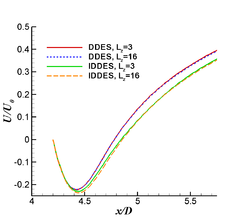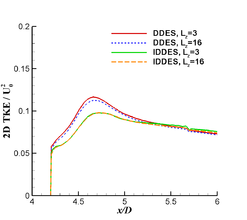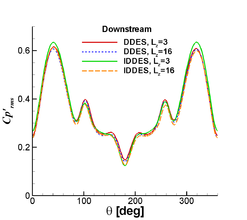Figure5: Effect of Lz/D on centreline distributions of mean velocity and 2D kinetic energy in the wake of the downstream cylinder (left and middle frames respectively) and on rms of pressure coefficient on the downstream cylinder (right) from incompressible SA DDES and SA IDDES of NTS.

Table 4: Effect of modelling approach and span-size of computational domain on the global shedding frequency.
Shedding frequency, Hz
DDES, Lz = 3D 188
DDES, Lz = 16D 188
IIDDES, Lz = 3D 192
IIDDES, Lz = 16D 192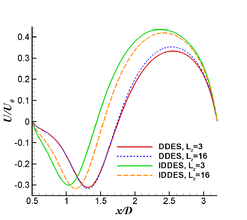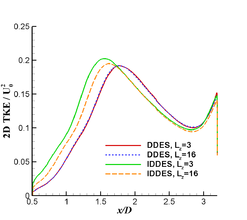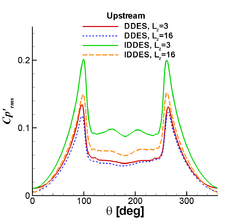Figure6: Effect of Lz/D on centreline distributions of mean velocity and 2D kinetic energy in the gap between cylinders (left and middle frames respectively) and on rms of pressure coefficient on the upstream cylinder (right) from incompressible SA DDES and IDDES of NTS.

1. The simulations at Lz/ D = 16 were conducted with the use of resources of the Argonne Leadership Computing Facility at Argonne National Laboratory, which is supported by the Office of Science of the U.S. Department of Energy under contract DE-AC02-06CH11357.

#### Compressibility effects

Considering that TUB simulations were carried out under assumption of incompressible flow and BTU and DLR performed compressible simulation at the experimental value of the Mach number (M = 0.128), it was important to find out how strongly this could affect the obtained results. In order to gain this knowledge NTS has carried out IDDES simulations both in the framework of incompressible and compressible problem statements. Results of these two simulations for the quantities which were found to be most sensitive to Lz/D (see Figure 6) are shown in Figure 7. They suggest that the role of the effects of compressibility in the considered flow is negligible.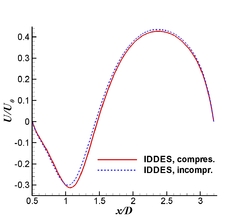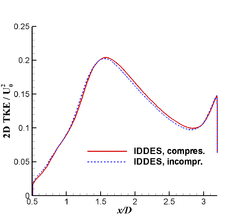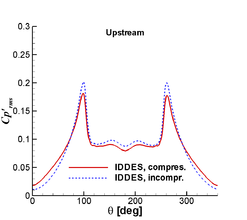Figure7: Comparison of results of SA IDDES at Lz/D = 3 carried out in incompressible and compressible (M = 0.128) modes. Left and middle frames: centreline distributions of mean velocity and 2D kinetic energy in the gap between cylinders; right frame: rms of pressure coefficient on the upstream cylinder.

#### Effect of time sample

This effect has been shown to be very strong for nominally 2D bodies with massive separation in many previous DES and LES studies. This is not surprising, since such flows typically have large scale coherent vortices in a vortex street pattern overlaid with finer random turbulent fluctuations at higher frequencies and random modulation and intermittency at frequencies lower than the vortex shedding one. This is true for the TC flow as well (see Figure 4), which dictates a need of rather long time samples for getting statistically representative mean flow characteristics and especially turbulence statistics. In order to exclude or at least minimize the effect of insufficient time sample when comparing results of different simulations, NTS has carried out a time-sample sensitivity study of the SA DDES of the TC flow. Its outcome is presented in Figure 8 and in Table 5. One can see that the time sample of about 150 convective time units (D / U0) is sufficient to obtain a reliable statistics not only for the mean drag but also for rms of the pressure coefficient. Exactly this value was recommended as a minimum one within the ATAAC project.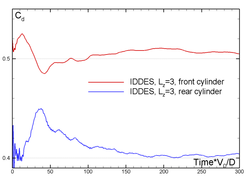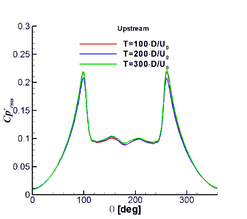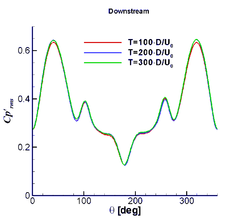Figure8: Effect of time sample on running time average of the drag coefficient (left frame) and rms of pressure coefficient on the surface of upstream (middle frame) and downstream (right frame) cylinders of tandem at Lz/D = 3 from incompressible SA IDDES

Table 5: Effect of time sample on time average of integral of TC configuration
Time, Convective time units (D/U0) CD upstream cylinder CD downstream cylinder
100 0.501 0.410
200 0.510 0.403
300 0.505 0.404

#### Effect of numerical dissipation

In order to evaluate this effect, NTS has carried compressible SA IDDES of the flow at Lz = 3D on the mandatory grid with the use of different approximations of the inviscid fluxes, Finv, available in the NTS code. All these approximations are based on the weighted upwind-biased (Fupw ) and central (Fctr ) schemes

Finv = (1 - σupw</sub> )  Fctr + σupw Fupw

with the solution-dependent empiric weight-function of the upwind scheme, σupw, computed as

σupw = max {σmax tanh(ACH1), σmin }

.

The function is designed so that σupw ∈ [σminσmax ] and is close to σmin in the LES region of DES and close to σmax in the RANS, irrotational, and departure regions . The series of simulations performed included three simulations, all carried out for compressible flow in free air rather than in the wind tunnel. The first and the second ones had σmin = 0 and combined the 4th order central scheme with 5th and 3rd order upwind-biased schemes, respectively, whereas the third one had σmin = 0.2 and combined 4th order central scheme with 3rd order upwind-biased scheme. Thus the first scheme has the minimum and the third scheme the maximum numerical dissipation with the second scheme somewhere in between . Some results from this series are presented in Figures 9, 10.

 Increase of dissipation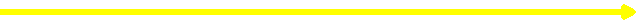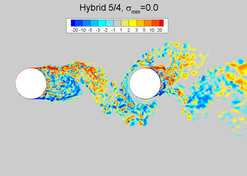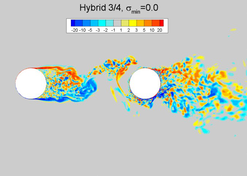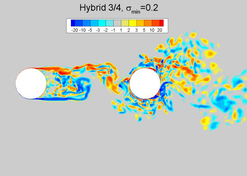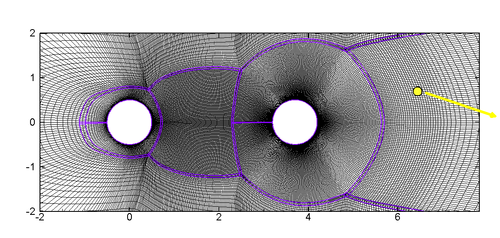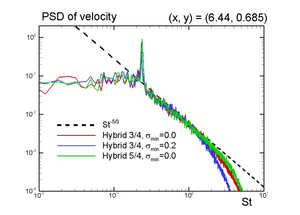Figure9: Effect of increase of numerical dissipation on snapshots of spanwise vortricity (upper row) and Power Spectral Density (PSD) of SA-IDDES streamwise velocity at a hot point in the wake of the downstream cylinder (lower row).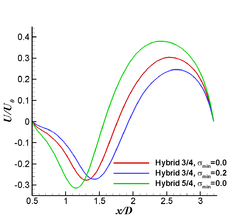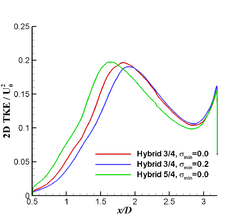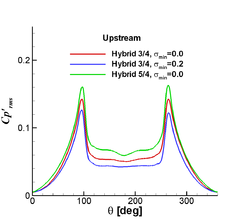Figure10: Effect of increase of numerical dissipation on centreline distributions of SA-IDDES mean velocity and 2D TKE in the gap between cylinders (left and middle frames) and on rms of pressure coefficient on the upstream cylinder (right frame).

They clearly show that increase of numerical dissipation causes a visible delay of the roll-up of the shear layer separated from the upstream cylinder and general damping of resolved turbulent structures (Figure 9). This, in turn, leads to a tangible variation not only of the unsteady pressure but also the mean flow parameters (Figure 10), which turns out to be comparable with their variation caused by the variation of the span size of the computational domain (see Figure 6 above). Based on these results, it can be concluded that using as low dissipative scheme as possible is essential for simulation of the considered and similar flows. Also, the fact that even relatively mild increase of the upwinding results in a considerable variation of the mean flow predictions suggests that the mandatory grid is not "fine-enough", which should be kept in mind when assessing agreement of results of simulations with the use of different turbulence modelling approaches with experiment presented in the comparison with experiment section below.

1. Note that all the NTS results presented in the comparison with experiment section below are obtained with the use of the first, less dissipative, scheme.

### COMPARISON WITH EXPERIMENT

In this section we present a comparison of predictions based on the simulations summarized in Table 3 above with experimental data 

Figure 11 presents flow visualizations from the PIV and from all the simulations in the form of instantaneous contours of the spanwise vorticity component. One can see that all the simulations predict qualitatively similar and generally plausible (consistent with the experiment) turbulent structures both in the gap between the cylinders and in the wake of the downstream cylinder. This suggests that the modelling approaches used, in principle, are capable of representing the key physics of the flow and that the numerical methods applied in all the codes possess essential features needed for turbulence- resolving simulations. At the same time, the comparison reveals considerable difference between the simulations which is most pronounced in the initial region of the free shear layers separated from the upstream cylinder, the peculiarities being associated with both turbulence models and codes.

We now move to a quantitative comparison with the experiment of the computed mean flow characteristics, particularly, distributions of mean pressure coefficient Cp over the surface of both cylinders and centreline distributions of the mean streamwise velocity in the gap between the cylinders and in the wake of the downstream cylinder.

As far as the pressure coefficient is concerned (see Figure 12), although the difference of the resolved turbulent structures described above naturally results in some difference of its predictions by different simulations, this effect turns out to be insignificant: all the computed distributions are, in general, reasonably close to each other and fairly well agree with the data, thus supporting a capability of a reliable prediction of the mean surface pressure by all the considered modelling approaches/codes. The only simulation in which prediction of the mean pressure is somewhat away from the other ones is that of SST DDES BTU. For the upstream cylinder it results in a bit too early separation and underestimation of the pressure peak but better than other simulations predicts a level of the pressure on its leeward part, whereas for the downstream cylinder, this level, on the contrary, turns out to be noticeably overestimated. DLR, NTS and TUB results (both SA DDES and SA IDDES) are very close to each other and generally agree well with the data.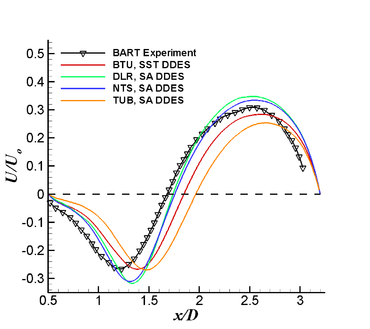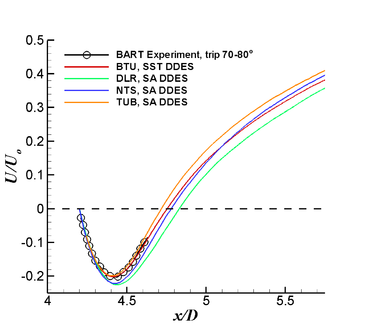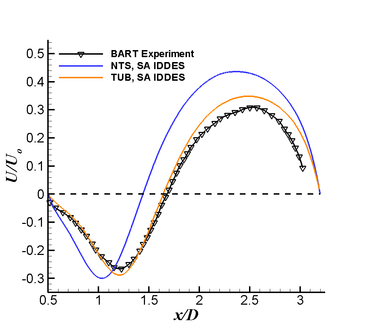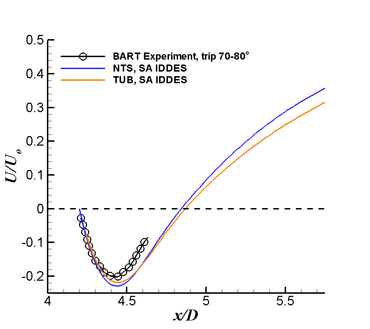Figure 13: Centerline distributions of mean streamwise velocity in the gap between cylinders (left column) and in the wake of the downstream cylinder (right column)

A comparison of centreline distributions of the mean streamwise velocity component, U /U0, shown in Figure 13 suggests that this flow quantity, especially in the gap between the cylinders (left column in the figure), turns out to be much more sensitive to both modelling approach and code, which is not surprising considering the strong sensitivity of this quantity to the numerics discussed above (see Figures 9, 10). In particular, within the DDES group (upper row in Figure 13), the length of the recirculation zone forming downstream of the upstream cylinder varies from about 1.65D (DLR and NTS SA DDES), which is very close to the experimental value, up to about 1.95D (TUB SA DDES), thus showing a considerable code-dependence. This is true also for the SA IDDES predictions of U /U0 in the NTS and TUB computations (see lower row in Figure 13). Both NTS and TUB observe the same model dependence between DDES and IDDES, namely a shortening of the recirculation length with IDDES. The magnitude of the effect is also similarly predicted by NTS and TUB. However, the degree of agreement with the benchmark data is predicted differently by these partners: For TUB, IDDES gives good agreement with the experiment, whereas for NTS the DDES approach is superior. This difference is most probably explained by the different level of numerical dissipation of numerics used in NTS and ELAN codes in the LES region of DDES and IDDES (central differences of the 4th and 2nd order for the inviscid fluxes respectively).

For the near wake of the downstream cylinder, the scatter of the predictions of the centreline velocity is somewhat reduced, and at x < 4.65D, where experimental data are available, they all are very close to the data.

A general conclusion regarding the mean flow prediction, which can be drawn based on the analysis of Figures 12 and 13, is that the surface pressure can be predicted by all the models and codes fairly well. For the velocity field, especially in the gap between cylinders, because of the strong code-sensitivity of the results, the situation is not that clear. Particularly, two of three SA DDES computations (those of DLR and NTS) predict close velocity distributions both well agreeing with the data. However, SA DDES of TUB gets quite a bit away from them. Other than that, for the same reason, according to NTS simulations, SA IDDES performs worse than SA DDES, whereas the SA IDDES solution of TUB turns out to be much closer to the data than their SA DDES. So, any absolute conclusions on the relative capabilities of DDES and IDDES, based on the analysis of U /U0 distributions in the gap regions remain elusive.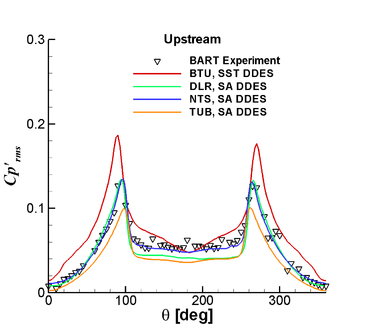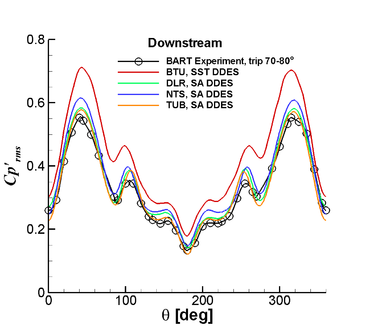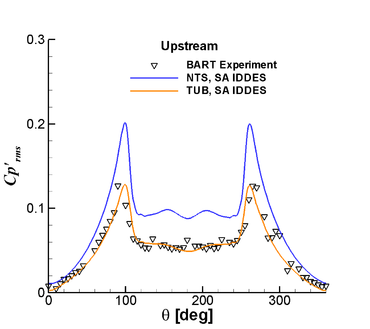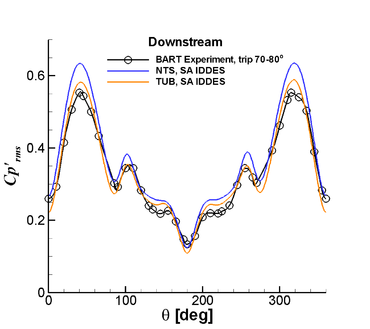Figure 14: Distributions of rms of pressure coefficient fluctuations on the upstream (left column) and downstream (right column) cylinders

Similar conclusions can be drawn regarding the unsteady flow characteristics which are of major interest in terms of aero-acoustic applications. In particular, as seen in Figure 14, the difference between distributions of the root-mean square (rms) of the surface pressure coefficient (C'p)rms caused by both different turbulence treatments and different numerics used in the simulations is rather large, especially for the upstream cylinder, where it reaches about a factor 1.5.

In the DDES group (upper row in Figure 14), the best prediction of this quantity is that of the SA based DDES of NTS: SA DDES of TUB underestimates it over all ϑ, SA DDES of DLR predicts too low level of (C'p)rms on the leeward part the cylinder, and SST DDES of BTU significantly overestimates (C'p)rms over the whole surface. For the downstream cylinder, good agreement with the data are obtained in all the simulations, except for the SST DDES of BTU, which tangibly overestimates (C'p)rms all over the cylinder. A possible explanation for the behaviour of the BTU simulation could be the earlier separation from the upstream cylinder, noted in the commentary to Fig. 12. This in turn, may be due to the underlying SST RANS model applied, however independent SST-based simulations with other codes would be required to confirm this. The earlier separation likely leads to a wider, more energetic wake, hence higher global levels of (C'p)rms. Both SA IDDES computations predict higher levels of (C'p)rms than their SA DDES counterparts (compare second and first rows in Figure 14), the trend being mostly pronounced for the upstream cylinder. This is consistent with the flow visualisations shown in Figure 11: IDDES predicts earlier roll-up of the shear layer separated from this cylinder than DDES. As a result, similar to what is observed for the centreline velocity distribution, SA IDDES of TUB gets very close to the data, whereas SA IDDES of NTS strongly overestimates (C'p)rms on the upstream cylinder and somewhat overestimates it on the downstream cylinder.

Figure 15 compares predicted and measured spectral characteristics of the surface pressure signals. It shows plots of Power Spectral Density (PSD) of the pressure fluctuations at the polar angle of 135° for the upstream (left column) and 45° for the downstream (right column) cylinders. One can see that the simulations reproduce major characteristics of the shape of experimental spectra, except for the plateau between 400Hz and 2000Hz observed in the experimental spectrum on the upstream cylinder, which is probably caused by the tripping of the boundary layers in the experiment (it was not observed in un-tripped configurations [2, 3]). Note also that SA DDES and SA IDDES spectra for the upstream cylinder of both NTS and TUB have high-frequency peaks (at about 1400Hz and 2000Hz with DDES and 2400Hz and 3000Hz with IDDES) which are not observed in the experimental spectra and are probably spurious (perhaps associated with an inadequate representation of the initial stage of the shear layer roll-up).

A quantitative comparison of the spectra reveals a rather wide scatter of frequencies and amplitudes of the primary spectral peak (see Table 6). This is consistent with the scatter of predicted locations of the separation on the upstream cylinder: an upstream shift of the separation should lead to slower shedding and lower frequencies. The trend is clearly seen in the SST DDES of BTU. Other than that, the spectral peak predicted by this simulation is for unknown reason more "smeared" compared with the other simulations and experiment. Finally, predicted levels of the broadband part of the spectrum for the upstream cylinder also turn out to be model- and code-dependent (the scatter of predictions reaches 10dB), whereas for the downstream cylinder these levels are close to each other and fairly well agree with the data.

Table6. Comparison of predicted and measured primary shredding frequencies tone's amplitudes
Primary shredding

frequency, Hz

Tone amplitude,

Upstream cylinder, dB

Tone amplitude,

Downstream cylinder, dB

BART Exp. 181 118.1 139.5
SA DDES, NTS 188.0 117.1 141.2
SA DDES, TUB 185.6 113.3 140.3
SST DDES, BTU 163.8 116.6 139.3
SA IDDES, NTS 192.7 124.3 142.7
SA IDDES, TUB 185.6 121.0 142.5

The scatter of the spectra from different simulations for the downstream cylinder is much less compared with the upstream cylinder, and all the predictions, except for those of the SST DDES of BTU, are in quite good agreement with the data (a tentative explanation for this based on the separation prediction by the SST model has been offered above). Considering that the input of the downstream cylinder in the noise generated by the tandem is dominant (the amplitude of the tone in the PSD of the pressure on the downstream cylinder is about 20dB larger than that on the upstream cylinder), this suggests that most of the simulations are capable of reliable prediction of the far-field noise of the tandem, which is a primary objective of turbulence-resolving simulations of the considered UFR.

One more characteristic of turbulence in the considered flow available in the experimental database is the two-dimensional (including only streamwise and lateral components of the velocity fluctuations) kinetic energy, 2D TKE. A comparison of the predicted and measured centreline distributions and profiles of this quantity in two flow sections is shown in Figures 16, 17 respectively. One can see that the scatter of its predictions is rather wide, none of the models being capable of capturing its experimental behaviour equally well in the whole flow.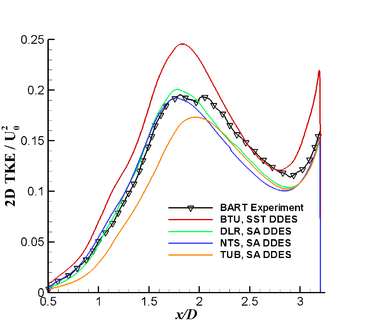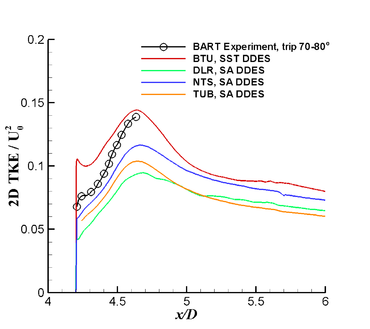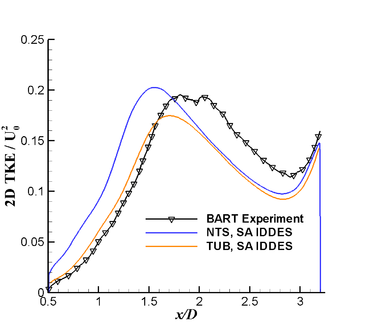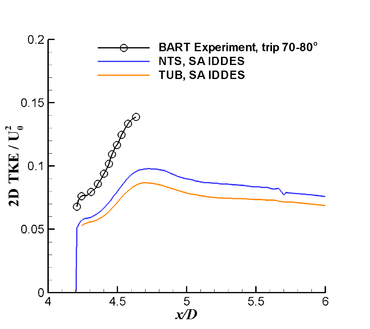Figure 16: Centerline distributions of resolved 2D TKE in the gap between cylinders (left column) and in the wake of the downstream cylinder (right column).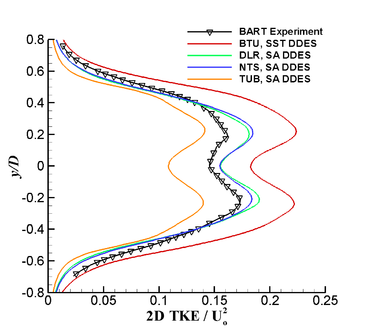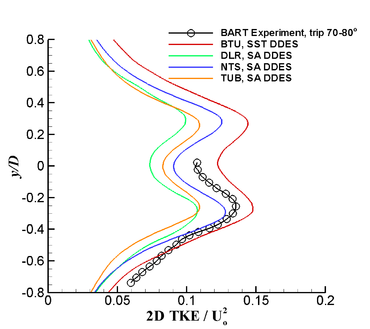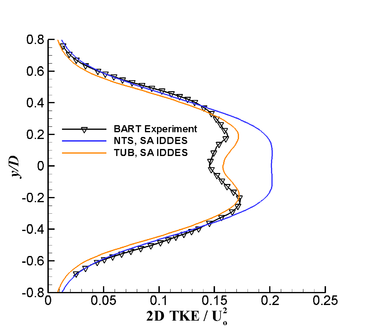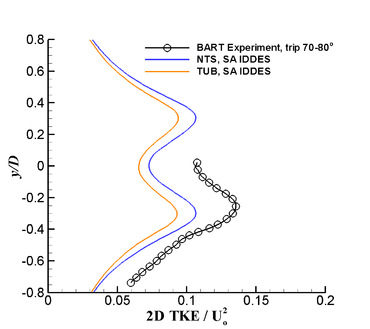Figure 17: Profiles of resolved 2D kinetic energy in the gap between cylinders at x = 1.5D (left column) and in the wake of the downstream cylinder at x = 4.5D (right column)

1. For NTS, results of the incompressible simulations are shown obtained at Lz = 3 with the use of hybrid, 5/4, scheme at σmin = 0.

Contributed by: A. Garbaruk, M. Shur and M. Strelets — New Technologies and Services LLC (NTS) and St.-Petersburg State Polytechnic University